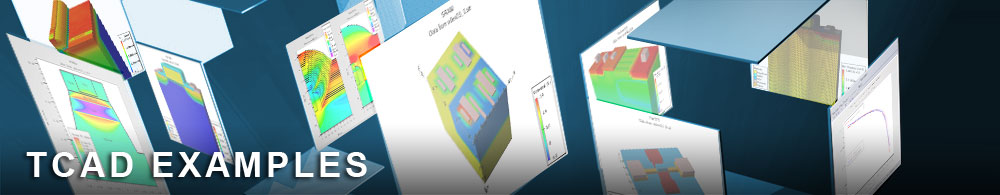Extraction of Hall scattering factors

## magneticex04.in : Extraction of Hall scattering factors

Requires: S-Pisces/Magnetic
Minimum Versions: Atlas 5.28.1.R

• Creating a 2d Hall cross structure
• How to set Hall scattering factors
• How to extract Hall scattering factors from simulation data

The Hall scattering factors, r_n and r_p, are theoretical constants which is put into MAGNETIC2D and affects the terms of the Magnetic matrix. For the limit n >> p we have a theoretical Hall coefficient of -r_n/n e and for p >> n we have a theoretical Hall coefficient of r_p/p e. The Hall coefficients can also be calculated directly from the simulated Hall voltage, current density and magnetic field as explained in the Atlas manual.

The purpose of this deck is to obtain the Hall coefficients directly from the simulation results, and multiply by the relevant carrier density to extract values for the hall scattering factors over a range of biases. These are then plotted to show that they agree closely with the values of Hall-scattering factor input on the MODELS statement. This acts as one validation of the Magnetic module.

Note: You should select anode bias as the X-quantity in the plot of the extracted data.

To load and run this example, select the Load button in DeckBuild > Examples. This will copy the input file and any support files to your current working directory. Select the Run button in DeckBuild to execute the example.